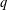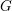Home

### Ring extensions and related topics (13Bxx)

• Article
• ##### ON SEPARABLE$\mathbb{A}^{2}$ AND$\mathbb{A}^{3}$ -FORMS
• Nagoya Mathematical Journal, First View

### Arithmetic rings and other special rings (13Fxx)

• Article
• #####$q$ -DEFORMED RATIONALS AND$q$ -CONTINUED FRACTIONS
• Forum of Mathematics, Sigma, Volume 8

### Differential algebra (13Nxx)

• Article
• ##### On the$K(\unicode[STIX]{x1D70B},1)$ -problem for restrictions of complex reflection arrangements
• Compositio Mathematica, Volume 156, Issue 3

• Article
• ##### ON EXISTENCE OF CANONICAL$G$ -BASES
• Nagoya Mathematical Journal, Volume 233

• Article
• ##### LOCALLY ANALYTIC REPRESENTATIONS OF$\text{GL}(2,L)$ VIA SEMISTABLE MODELS OF$\mathbb{P}^{1}$
• Journal of the Institute of Mathematics of Jussieu, Volume 18, Issue 1